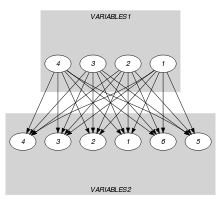## 5.78. common_partition

Origin

Derived from $\mathrm{𝚌𝚘𝚖𝚖𝚘𝚗}$.

Constraint

$\mathrm{𝚌𝚘𝚖𝚖𝚘𝚗}_\mathrm{𝚙𝚊𝚛𝚝𝚒𝚝𝚒𝚘𝚗}\left(\begin{array}{c}\mathrm{𝙽𝙲𝙾𝙼𝙼𝙾𝙽}\mathtt{1},\hfill \\ \mathrm{𝙽𝙲𝙾𝙼𝙼𝙾𝙽}\mathtt{2},\hfill \\ \mathrm{𝚅𝙰𝚁𝙸𝙰𝙱𝙻𝙴𝚂}\mathtt{1},\hfill \\ \mathrm{𝚅𝙰𝚁𝙸𝙰𝙱𝙻𝙴𝚂}\mathtt{2},\hfill \\ \mathrm{𝙿𝙰𝚁𝚃𝙸𝚃𝙸𝙾𝙽𝚂}\hfill \end{array}\right)$

Type
 $\mathrm{𝚅𝙰𝙻𝚄𝙴𝚂}$ $\mathrm{𝚌𝚘𝚕𝚕𝚎𝚌𝚝𝚒𝚘𝚗}\left(\mathrm{𝚟𝚊𝚕}-\mathrm{𝚒𝚗𝚝}\right)$
Arguments
 $\mathrm{𝙽𝙲𝙾𝙼𝙼𝙾𝙽}\mathtt{1}$ $\mathrm{𝚍𝚟𝚊𝚛}$ $\mathrm{𝙽𝙲𝙾𝙼𝙼𝙾𝙽}\mathtt{2}$ $\mathrm{𝚍𝚟𝚊𝚛}$ $\mathrm{𝚅𝙰𝚁𝙸𝙰𝙱𝙻𝙴𝚂}\mathtt{1}$ $\mathrm{𝚌𝚘𝚕𝚕𝚎𝚌𝚝𝚒𝚘𝚗}\left(\mathrm{𝚟𝚊𝚛}-\mathrm{𝚍𝚟𝚊𝚛}\right)$ $\mathrm{𝚅𝙰𝚁𝙸𝙰𝙱𝙻𝙴𝚂}\mathtt{2}$ $\mathrm{𝚌𝚘𝚕𝚕𝚎𝚌𝚝𝚒𝚘𝚗}\left(\mathrm{𝚟𝚊𝚛}-\mathrm{𝚍𝚟𝚊𝚛}\right)$ $\mathrm{𝙿𝙰𝚁𝚃𝙸𝚃𝙸𝙾𝙽𝚂}$ $\mathrm{𝚌𝚘𝚕𝚕𝚎𝚌𝚝𝚒𝚘𝚗}\left(𝚙-\mathrm{𝚅𝙰𝙻𝚄𝙴𝚂}\right)$
Restrictions
 $|\mathrm{𝚅𝙰𝙻𝚄𝙴𝚂}|\ge 1$ $\mathrm{𝚛𝚎𝚚𝚞𝚒𝚛𝚎𝚍}$$\left(\mathrm{𝚅𝙰𝙻𝚄𝙴𝚂},\mathrm{𝚟𝚊𝚕}\right)$ $\mathrm{𝚍𝚒𝚜𝚝𝚒𝚗𝚌𝚝}$$\left(\mathrm{𝚅𝙰𝙻𝚄𝙴𝚂},\mathrm{𝚟𝚊𝚕}\right)$ $\mathrm{𝙽𝙲𝙾𝙼𝙼𝙾𝙽}\mathtt{1}\ge 0$ $\mathrm{𝙽𝙲𝙾𝙼𝙼𝙾𝙽}\mathtt{1}\le |\mathrm{𝚅𝙰𝚁𝙸𝙰𝙱𝙻𝙴𝚂}\mathtt{1}|$ $\mathrm{𝙽𝙲𝙾𝙼𝙼𝙾𝙽}\mathtt{2}\ge 0$ $\mathrm{𝙽𝙲𝙾𝙼𝙼𝙾𝙽}\mathtt{2}\le |\mathrm{𝚅𝙰𝚁𝙸𝙰𝙱𝙻𝙴𝚂}\mathtt{2}|$ $\mathrm{𝚛𝚎𝚚𝚞𝚒𝚛𝚎𝚍}$$\left(\mathrm{𝚅𝙰𝚁𝙸𝙰𝙱𝙻𝙴𝚂}\mathtt{1},\mathrm{𝚟𝚊𝚛}\right)$ $\mathrm{𝚛𝚎𝚚𝚞𝚒𝚛𝚎𝚍}$$\left(\mathrm{𝚅𝙰𝚁𝙸𝙰𝙱𝙻𝙴𝚂}\mathtt{2},\mathrm{𝚟𝚊𝚛}\right)$ $\mathrm{𝚛𝚎𝚚𝚞𝚒𝚛𝚎𝚍}$$\left(\mathrm{𝙿𝙰𝚁𝚃𝙸𝚃𝙸𝙾𝙽𝚂},𝚙\right)$ $|\mathrm{𝙿𝙰𝚁𝚃𝙸𝚃𝙸𝙾𝙽𝚂}|\ge 2$
Purpose

$\mathrm{𝙽𝙲𝙾𝙼𝙼𝙾𝙽}\mathtt{1}$ is the number of variables of the $\mathrm{𝚅𝙰𝚁𝙸𝙰𝙱𝙻𝙴𝚂}\mathtt{1}$ collection taking a value in a partition derived from the values assigned to the variables of $\mathrm{𝚅𝙰𝚁𝙸𝙰𝙱𝙻𝙴𝚂}\mathtt{2}$ and from $\mathrm{𝙿𝙰𝚁𝚃𝙸𝚃𝙸𝙾𝙽𝚂}$.

$\mathrm{𝙽𝙲𝙾𝙼𝙼𝙾𝙽}\mathtt{2}$ is the number of variables of the $\mathrm{𝚅𝙰𝚁𝙸𝙰𝙱𝙻𝙴𝚂}\mathtt{2}$ collection taking a value in a partition derived from the values assigned to the variables of $\mathrm{𝚅𝙰𝚁𝙸𝙰𝙱𝙻𝙴𝚂}\mathtt{1}$ and from $\mathrm{𝙿𝙰𝚁𝚃𝙸𝚃𝙸𝙾𝙽𝚂}$.

Example
$\left(\begin{array}{c}3,4,〈2,3,6,0〉,\hfill \\ 〈0,6,3,3,7,1〉,\hfill \\ 〈𝚙-〈1,3〉,𝚙-〈4〉,𝚙-〈2,6〉〉\hfill \end{array}\right)$

In the example, the last argument $\mathrm{𝙿𝙰𝚁𝚃𝙸𝚃𝙸𝙾𝙽𝚂}$ defines the partitions $𝚙-〈1,3〉$, $𝚙-〈4〉$ and $𝚙-〈2,6〉$. As a consequence the first three items of collection $〈2,3,6,0〉$ respectively correspond to the partitions $𝚙-〈2,6〉$, $𝚙-〈1,3〉$, and $𝚙-〈2,6〉$. Similarly the items of collection $〈0,6,3,3,7,1〉$ (from which we remove items 0 and 7 since they do not belong to any partition) respectively correspond to the partitions $𝚙-〈2,6〉$, $𝚙-〈1,3〉$, $𝚙-〈1,3〉$, and $𝚙-〈1,3〉$. The $\mathrm{𝚌𝚘𝚖𝚖𝚘𝚗}_\mathrm{𝚙𝚊𝚛𝚝𝚒𝚝𝚒𝚘𝚗}$ constraint holds since:

• Its first argument $\mathrm{𝙽𝙲𝙾𝙼𝙼𝙾𝙽}\mathtt{1}=3$ is the number of partitions associated with the items of collection $〈2,3,6,0〉$ that also correspond to partitions associated with $〈0,6,3,3,7,1〉$.

• Its second argument $\mathrm{𝙽𝙲𝙾𝙼𝙼𝙾𝙽}\mathtt{2}=4$ is the number of partitions associated with the items of collection $〈0,6,3,3,7,1〉$ that also correspond to partitions associated with $〈2,3,6,0〉$.

Typical
 $|\mathrm{𝚅𝙰𝚁𝙸𝙰𝙱𝙻𝙴𝚂}\mathtt{1}|>1$ $\mathrm{𝚛𝚊𝚗𝚐𝚎}$$\left(\mathrm{𝚅𝙰𝚁𝙸𝙰𝙱𝙻𝙴𝚂}\mathtt{1}.\mathrm{𝚟𝚊𝚛}\right)>1$ $|\mathrm{𝚅𝙰𝚁𝙸𝙰𝙱𝙻𝙴𝚂}\mathtt{2}|>1$ $\mathrm{𝚛𝚊𝚗𝚐𝚎}$$\left(\mathrm{𝚅𝙰𝚁𝙸𝙰𝙱𝙻𝙴𝚂}\mathtt{2}.\mathrm{𝚟𝚊𝚛}\right)>1$ $|\mathrm{𝚅𝙰𝚁𝙸𝙰𝙱𝙻𝙴𝚂}\mathtt{1}|>|\mathrm{𝙿𝙰𝚁𝚃𝙸𝚃𝙸𝙾𝙽𝚂}|$ $|\mathrm{𝚅𝙰𝚁𝙸𝙰𝙱𝙻𝙴𝚂}\mathtt{2}|>|\mathrm{𝙿𝙰𝚁𝚃𝙸𝚃𝙸𝙾𝙽𝚂}|$
Symmetries
• Arguments are permutable w.r.t. permutation $\left(\mathrm{𝙽𝙲𝙾𝙼𝙼𝙾𝙽}\mathtt{1},\mathrm{𝙽𝙲𝙾𝙼𝙼𝙾𝙽}\mathtt{2}\right)$ $\left(\mathrm{𝚅𝙰𝚁𝙸𝙰𝙱𝙻𝙴𝚂}\mathtt{1},\mathrm{𝚅𝙰𝚁𝙸𝙰𝙱𝙻𝙴𝚂}\mathtt{2}\right)$ $\left(\mathrm{𝙿𝙰𝚁𝚃𝙸𝚃𝙸𝙾𝙽𝚂}\right)$.

• Items of $\mathrm{𝚅𝙰𝚁𝙸𝙰𝙱𝙻𝙴𝚂}\mathtt{1}$ are permutable.

• Items of $\mathrm{𝚅𝙰𝚁𝙸𝙰𝙱𝙻𝙴𝚂}\mathtt{2}$ are permutable.

• Items of $\mathrm{𝙿𝙰𝚁𝚃𝙸𝚃𝙸𝙾𝙽𝚂}$ are permutable.

• Items of $\mathrm{𝙿𝙰𝚁𝚃𝙸𝚃𝙸𝙾𝙽𝚂}.𝚙$ are permutable.

• An occurrence of a value of $\mathrm{𝚅𝙰𝚁𝙸𝙰𝙱𝙻𝙴𝚂}\mathtt{1}.\mathrm{𝚟𝚊𝚛}$ can be replaced by any other value that also belongs to the same partition of $\mathrm{𝙿𝙰𝚁𝚃𝙸𝚃𝙸𝙾𝙽𝚂}$.

• An occurrence of a value of $\mathrm{𝚅𝙰𝚁𝙸𝙰𝙱𝙻𝙴𝚂}\mathtt{2}.\mathrm{𝚟𝚊𝚛}$ can be replaced by any other value that also belongs to the same partition of $\mathrm{𝙿𝙰𝚁𝚃𝙸𝚃𝙸𝙾𝙽𝚂}$.

Arg. properties
• Functional dependency: $\mathrm{𝙽𝙲𝙾𝙼𝙼𝙾𝙽}\mathtt{1}$ determined by $\mathrm{𝚅𝙰𝚁𝙸𝙰𝙱𝙻𝙴𝚂}\mathtt{1}$, $\mathrm{𝚅𝙰𝚁𝙸𝙰𝙱𝙻𝙴𝚂}\mathtt{2}$ and $\mathrm{𝙿𝙰𝚁𝚃𝙸𝚃𝙸𝙾𝙽𝚂}$.

• Functional dependency: $\mathrm{𝙽𝙲𝙾𝙼𝙼𝙾𝙽}\mathtt{2}$ determined by $\mathrm{𝚅𝙰𝚁𝙸𝙰𝙱𝙻𝙴𝚂}\mathtt{1}$, $\mathrm{𝚅𝙰𝚁𝙸𝙰𝙱𝙻𝙴𝚂}\mathtt{2}$ and $\mathrm{𝙿𝙰𝚁𝚃𝙸𝚃𝙸𝙾𝙽𝚂}$.

specialisation: $\mathrm{𝚌𝚘𝚖𝚖𝚘𝚗}$ ($\mathrm{𝚟𝚊𝚛𝚒𝚊𝚋𝚕𝚎}\in \mathrm{𝚙𝚊𝚛𝚝𝚒𝚝𝚒𝚘𝚗}$ replaced by $\mathrm{𝚟𝚊𝚛𝚒𝚊𝚋𝚕𝚎}$).

Keywords
Arc input(s)

$\mathrm{𝚅𝙰𝚁𝙸𝙰𝙱𝙻𝙴𝚂}\mathtt{1}$ $\mathrm{𝚅𝙰𝚁𝙸𝙰𝙱𝙻𝙴𝚂}\mathtt{2}$

Arc generator
$\mathrm{𝑃𝑅𝑂𝐷𝑈𝐶𝑇}$$↦\mathrm{𝚌𝚘𝚕𝚕𝚎𝚌𝚝𝚒𝚘𝚗}\left(\mathrm{𝚟𝚊𝚛𝚒𝚊𝚋𝚕𝚎𝚜}\mathtt{1},\mathrm{𝚟𝚊𝚛𝚒𝚊𝚋𝚕𝚎𝚜}\mathtt{2}\right)$

Arc arity
Arc constraint(s)
$\mathrm{𝚒𝚗}_\mathrm{𝚜𝚊𝚖𝚎}_\mathrm{𝚙𝚊𝚛𝚝𝚒𝚝𝚒𝚘𝚗}$$\left(\mathrm{𝚟𝚊𝚛𝚒𝚊𝚋𝚕𝚎𝚜}\mathtt{1}.\mathrm{𝚟𝚊𝚛},\mathrm{𝚟𝚊𝚛𝚒𝚊𝚋𝚕𝚎𝚜}\mathtt{2}.\mathrm{𝚟𝚊𝚛},\mathrm{𝙿𝙰𝚁𝚃𝙸𝚃𝙸𝙾𝙽𝚂}\right)$
Graph property(ies)
 $•$$\mathrm{𝐍𝐒𝐎𝐔𝐑𝐂𝐄}$$=\mathrm{𝙽𝙲𝙾𝙼𝙼𝙾𝙽}\mathtt{1}$ $•$$\mathrm{𝐍𝐒𝐈𝐍𝐊}$$=\mathrm{𝙽𝙲𝙾𝙼𝙼𝙾𝙽}\mathtt{2}$

Graph class
 $•$$\mathrm{𝙰𝙲𝚈𝙲𝙻𝙸𝙲}$ $•$$\mathrm{𝙱𝙸𝙿𝙰𝚁𝚃𝙸𝚃𝙴}$ $•$$\mathrm{𝙽𝙾}_\mathrm{𝙻𝙾𝙾𝙿}$

Graph model

Parts (A) and (B) of Figure 5.78.1 respectively show the initial and final graph associated with the Example slot. Since we use the $\mathrm{𝐍𝐒𝐎𝐔𝐑𝐂𝐄}$ and $\mathrm{𝐍𝐒𝐈𝐍𝐊}$ graph properties, the source and sink vertices of the final graph are stressed with a double circle. Since the graph has only 3 sources and 4 sinks the variables $\mathrm{𝙽𝙲𝙾𝙼𝙼𝙾𝙽}\mathtt{1}$ and $\mathrm{𝙽𝙲𝙾𝙼𝙼𝙾𝙽}\mathtt{2}$ are respectively equal to 3 and 4. Note that the vertices corresponding to the variables that take values 0 or 7 were removed from the final graph since there is no arc for which the associated $\mathrm{𝚒𝚗}_\mathrm{𝚜𝚊𝚖𝚎}_\mathrm{𝚙𝚊𝚛𝚝𝚒𝚝𝚒𝚘𝚗}$ constraint holds.

##### Figure 5.78.1. Initial and final graph of the $\mathrm{𝚌𝚘𝚖𝚖𝚘𝚗}_\mathrm{𝚙𝚊𝚛𝚝𝚒𝚝𝚒𝚘𝚗}$ constraint(a) (b)